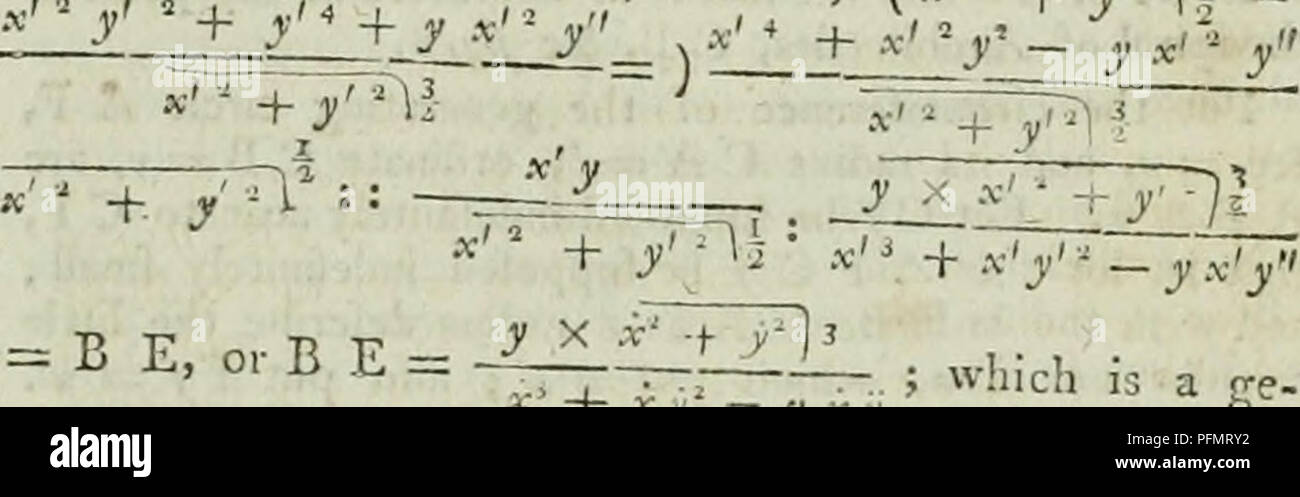# . The cyclopaedia; or, Universal dictionary of arts, sciences, and literature. Encyclopedias and dictionaries. equation (the fluxion of y being negative), pyy â f zay-ây.g a â¢ J- â¢ 2 a j' - j--&quot; â 'â (by writing for y its equal), ; f Now, by fubftituting â-ââ 2 12 that &quot;^^ for v% and â for y, f â¢we have ' + . 2 a BÂ« = x1, and n b = y, when, by 47 E. 1 B b -s *' V ^* . â will be = x'''+' Â»i*iJ *' &quot; + y' = B F or B ;â¢ ; and B b I b. n y y' + y rs&gt;, y +/&quot; : v :â-= -, , x'' y ri : : B C : C F, that is, = C F or C r ; the in- crement of which is rfj that i## Image details

Contributor:

Central Historic Books / Alamy Stock Photo

Image ID:

PFMRY2

File size:

Releases:

Model - no | Property - noDo I need a release?

Dimensions:

2826 x 885 px | 23.9 x 7.5 cm | 9.4 x 3 inches | 300dpi

This image is a public domain image, which means either that copyright has expired in the image or the copyright holder has waived their copyright. Alamy charges you a fee for access to the high resolution copy of the image.

This image could have imperfections as it’s either historical or reportage.

. The cyclopaedia; or, Universal dictionary of arts, sciences, and literature. Encyclopedias and dictionaries. equation (the fluxion of y being negative), pyy â f zay-ây.g _ a â¢ J- â¢ 2 a j' - j--&quot; â 'â (by writing for _y its equal), ; f Now, by fubftituting â-ââ 2 12 that &quot;^^ for v% and â for y, f â¢we have ' + . 2 a BÂ« = x1, and n b = y, when, by 47 E. 1 B b -s *' V ^* . â will be = x'''+' Â»i~*iJ *' &quot; + y' = B F or B ;â¢ ; and B b I b. n y y' + y rs&gt;, y +/&quot; : v :â-= -, , x'' y ri : : B C : C F, that is, = C F or C r ; the in- crement of which is rfj that is, fuppofmg x' to be inva- y&quot; -i-yy&quot; x x7* +/â â ] i - y'y'xjy'^ riable) , *ZZZHi__ _ 2y&quot; +/* + yÂ«&quot;y&quot; ^TT - rf. Again, the triangles E Â£ b and E r / being fimilar, B b - r f: R b â â¢ (BE - r E, or) r B : B E ; that is, (Â«fÂ« +.y/)| _. neral expreffion for the radius of curvature of all curves referred to a fixed or central point, when *,' or x is inva- riable. Hence, if .v be made =r 1, the general expreffion for the Wliere- radius of curvature will be -^^ I ^ ^_] fore, if we put the equation of the given fpiral into fluxions, making ^? = ,, and put this fluxional equation into fluxions again; and from thence, or from the na- ture of the curve, find the values of i&quot; and y : then, if for;- and y we fubftitute thefe their values in this ffene- ralexprelhon, we ihail have B E the radius tif curvature re- quired. Otherwife. Let A R B he the propofedcui-veyiftfe - ) P the point, or centre, to which its ordinates are referred. JN O L the evolute, and R O the ray of curvature at R Moreover let P H be perpendicular 'to R O ; an,', fuppofl jng the ordinate PR. (y) to I )tion of the point K alon fA Rand P H (/.), exprefling the eel . , jn1 , ;&gt;_ aâd H '? dlreÂ«K&quot; '' n ir to R O, be denoted by i and p refpeftiyely : the flu do s if quantitii 1 b the celerities by wl I in magnitude. (S;, ion), Therel re 1 celer

Save up to 30% with our image packs